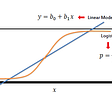# Singular Vector Decomposition in Numpy, Python

From the previous posting, we have learned how to do SVD, Singular Vector Decomposition with great examples and illustrations. So, today we will go over the material with python coding taking a practical approach toward it so that we can use it right away after reading this posting. Let’s get started!

So, we have a goal set, just below, and will work on two equations, 1 and 2 to accomplish SVD.

First of all, in Python, I will load Numpy library and declare a matrix (which would be a numpy array) under the name ‘A’.

`import numpy as npA = np.array([[5,5],             [-1, 7]])`

A is a 2x2 matrix. Not, let’s declare A’s transpose matrix under the name ‘A_transpose’.

`A_transpose = A.T# A_transpose = A.transpose()`

“.T” is a method function inside Numpy library. Also, you can use “.transpose()” which does the exact same job.

Now, we will make the first equation condition, and store the matrix multiplication value under the name ‘eq_1’.

`eq_1 = np.matmul(A_transpose,A)`

We can easily multiply a number of matrices’ multiplication with Numpy’s innate function ‘matmul()’.

And we can check the value of eq_1 by printing it out. (below)

Now, we will go another step calculating eigenvalues of ‘eq_1’ matrix, and getting the value for the matrix V.

Getting eigenvalues is pretty easy since what we need to do is calling a function in Numpy, Numpy.linalg.eig().

`eigen_value_1, eigen_value_2 = np.linalg.eig(eq_1)`

And two eigenvalues are stored in two different names, one is in “eigen_value_1”, and the other is “eigen_value_2”. Let’s print them out!

The order between the two does not matter. We can also store 80 in 1st eigenvalue too.

Next, let’s check each of the results that subtracting “eigenvalue multiplied identity matrix” from our ‘eq_1’.

For the identity matrix, we can use numpy.eye(). (Sounds same, eye, and I from identity)

`c = eq_1- eigen_value_1*np.eye(2)d = eq_1-eigen_value_2*np.eye(2)`

From c, and d, we can find the vector that would consist V.

In ‘c’, we can see that if we multiply the first row [6, 18] by (-3) and adding it to the 2nd row [18, 54], then it would be zero. This would be ingredients to make “V_1”.

In ‘d’, we can see that multiplying the second row with 3 and adding it to the first row would make it zero. And “V_2” will come out with this result.

`V_1 = np.array([-3/np.sqrt(10), 1/np.sqrt(10)])V_2 = np.array([1/np.sqrt(10), 3/np.sqrt(10)])V = np.vstack([V_1, V_2])`

And, our “S” would be make of two “square-root eigenvalues” in diagonal direction and zero other

`S = np.array([[np.sqrt(20),0],[0, np.sqrt(80)]])`

As we have now have S and V, the only thing left to get is U.

And based on this, we can get it by multiplying A, V, and inverse matrix of S.

`S_inv = np.linalg.inv(S)U = np.matmul(AV, S_inv)`

Actually there are way easier method to earn these values. Numpy supports SVD by Numpy.linalg.svd().

Try that out, and also try to implement step by step to get each value for the Singular Vector Decomposition. To tell you the truth, I learn this by doing this. As you know already, learning by doing!

See you later :) and stay tuuuuunnned!

--

--

--

Ydobon is nobody.

Love podcasts or audiobooks? Learn on the go with our new app.

## Workflow of a Machine Learning Project## Decentralized supervised neural network on the Blockchain: Giving mining a good purpose## How to choose the right type of machine learning algorithm? (Part 1)## Machine learning scholar adventure: Chapter 1## Difference between Supervised and Unsupervised Learning## Confusion Matrix, ROC_AUC and Imbalanced Classes in Logistic Regression## Machine Learning in Predicting Stock Market States## A Ydobon

Ydobon is nobody.

## Logistic Regression — General Overview## Fake News Detection using Machine Learning models## Machine Learning — Data Analysis## ML-Support Vector Machine